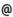## Babes-Bolyai University of Cluj-Napoca Faculty of Mathematics and Computer Science Study Cycle: Graduate SUBJECT

 Code Subject MMN0001 Mathematical Software
 Section Semester Hours: C+S+L Category Type Mathematics 3 2+0+2 speciality compulsory Applied Mathematics 3 2+0+2 speciality compulsory
 Teaching Staff in Charge
 Assoc.Prof. TRÎMBITAS Radu Tiberiu, Ph.D.,  tradumath.ubbcluj.roAssoc.Prof. SOOS Anna, Ph.D.,  asoosmath.ubbcluj.roLect. LISEI Hannelore-Inge, Ph.D.,  hannemath.ubbcluj.ro
 Aims To initiate students in using mathematical software and typewriting mathematical texts
 Content - Introduction into MATLAB - MATLAB environment, features, basic types, interaction(1 Lecture) - Matrices in MATLAB - generating, indexing, matrix and array operations(1 Lecture) - MATLAB programming- control flows, M files, data types, advanced data structures (1 Lecture) - MATLAB Graphics ( 3 Lectures) - 2D graphics, 3D graphics, volume visualization, animation, GUIs - Mathematics in MATLAB Numerical linear algebra (1 Lecture) ODE solvers (1 Lecture) Data Analysis (1 Lecture) Symbolic computation(1 Lecture) - Introduction into MAPLE (2 Lectures) - LaTeX (2 Lectures) Text formating, spacing, classes and styles Math formulas, floating bodies, graph insertion in LaTeX Packages, Web resources
 References P. Blaga: Statistica prin MATLAB, Presa Universitara Clujeana, 2003 The Mathworks - Setul de manuale MATLAB Cleve Moler - Numerical Computing in MATLAB, SIAM, 2005 D. J. Higham, N. J. Higham, MATLAB Guide, 2nd edition, SIAM, 2005 Radu Trimbitas - Analiza numerica. O introducere bazata pe MATLAB, Presa Universitara Clujeana Horia F. Pop, Paul A. Blaga - LaTex -2e, Editura Tehnica, Bucuresti, 1999 P. Marchand, O. T. Holand - Graphics and GUI with MATLAB, 3rd edition, Barnes and Noble, 2003
 Assessment Practical test - 75%, semester activity - 25%
 Links: Syllabus for all subjects Romanian version for this subject Rtf format for this subject